EXPERIMENT NO.5

TITLE: FREQUENCY RESPONSE OF TWIN-T NETWORK

THEORY:

Twin-T Network

Using z parameter to describe a two port network:

There are number of methods available for analytical describing linear time invariant electrical network. These include parameterization by Z, Y, H, G, ABCD (chain matrix) and S parameters. Network to be analyzed and constructed will be in audio frequency range hence classical Z parameters are easy to measure and will be used. Any of other representation will be obtained by appropriate linear dimension including S parameters.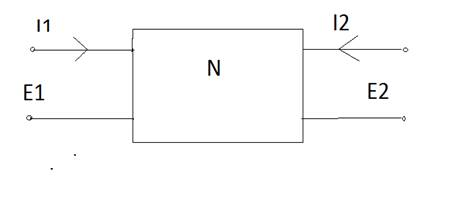Fig-Ganeral Two Port Network

Matrix equation for Z parameters is:=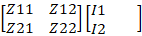These Zâ€™s are called open circut impedence,since typically output or input p[ort current is force to zero (open circulated)to evaluate parameter shown in equation.

Z11=Â½I2=0 ; E21=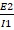Â½I2=0

Z12=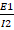Â½I1=0 ; Z22=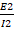Â½I1=0

Constructing Test circuit:

This circuit can be constructed using readily available component. The above analysis assumed ideal zero tolerance components and clearly the agreement between the analysis and measurement will be determine by the tolerance of component for best result use good quality polystyrene capacitor. Ceramic capacitor with high dielectric constant are often non linear and should be avoided.

For positive network like the Twin-T at most 3 of four parameters are independent The reciprocity theorem state that the transfer function of positive network with dimension of impedance (or admittance) remain unchanged if the point of excitation and response are unchanged. The theorem demands that the transfer impedance Z21 and Z12 must be equal.

Furthermore, if the network is symmetrical with respect to the input and output port as in the Twin-T, Z22 must be equal to Z11.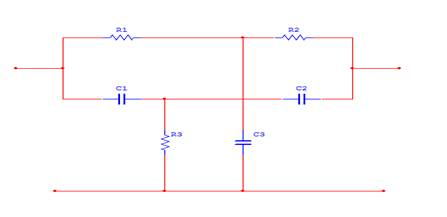Fig-Twin-T Network

R1=R2=R;

C1=C2=C;

R3=R/2;

C3=2C.

Analysis of Twin-T yield:

Z11 =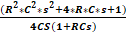Z21 =E21 =Â½(I2=0) ==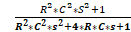The following MATLAB script evaluates above equation along jw axis over the dc to 10 KHz region. As can be seen from script the analysis uses the component value given in above port list .The script compute Z11 and Z12 for subsequent use and plot is made of the voltage transfer function X21.To run the script simply point and click anywhere in green MATLAB script, and then press MATLAB eval batten on tool bar below. The script will execute and a plot of magnitude of X21 Vs Frequency will be made.

MATLAB CODE

%Frequency responce of Twin-t network

f=0:25:10000;

jw=2*pi*f*j;

R=1000;

C=0.047e-6;

a=polyval([R^2*C^2,4*R*C,1],jw);

b=polyval([R^2*C^2,0,1],jw);

c=eps+polyval(4*C*[R*C,1,10],jw);

z11=a./c;

z12=b./c;

X21=b./a;

plot(f,20*log10(abs(X21)),'b');

title('TWIN T NETWORK FREQUENCY RESPONCE');

xlabel('HERTZS');

ylebel('dB');

OUTPUT: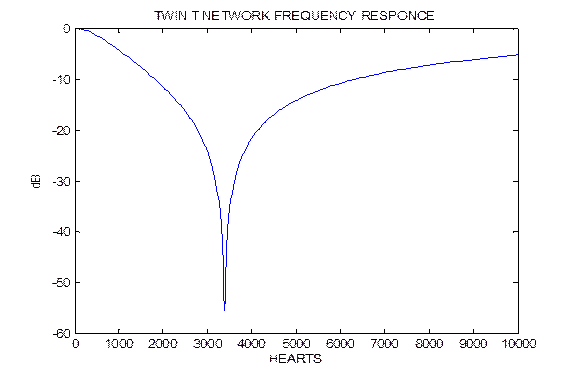Fig-Computed Twin-T voltage Transfer Function

CONCLUSION: